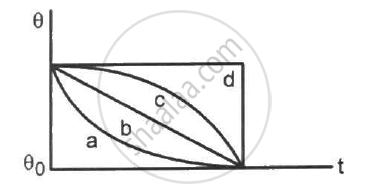Department of Pre-University Education, KarnatakaPUC Karnataka Science Class 11

# A Hot Liquid is Kept in a Big Room. Its Temperature is Plotted as a Function of Time. Which of the Following Curves May Represent the Plot? - Physics

MCQ

A hot liquid is kept in a big room. Its temperature is plotted as a function of time. Which of the following curves may represent the plot?• a

• b

• c

• d

#### Solution

a

When a hot liquid is kept in a big room, the liquid will loose its temperature with time. The thermal energy emitted by the liquid will be gained by the walls of the room. As the room is big, we can assume that the temperature difference between the room and the liquid is large. From Stephen's law, the liquid emits thermal energy in proportion to T^4, where T is the initial temperature of the liquid.
As the temperature decreases, the rate of loss of thermal energy will also decrease. So, the slope of the curve will also decrease.
Therefore, the plot of temperature with time is best represented by the curve (a).

Concept: Stefan's Law
Is there an error in this question or solution?

#### APPEARS IN

HC Verma Class 11, Class 12 Concepts of Physics Vol. 2
Chapter 6 Heat Transfer
MCQ | Q 8 | Page 97